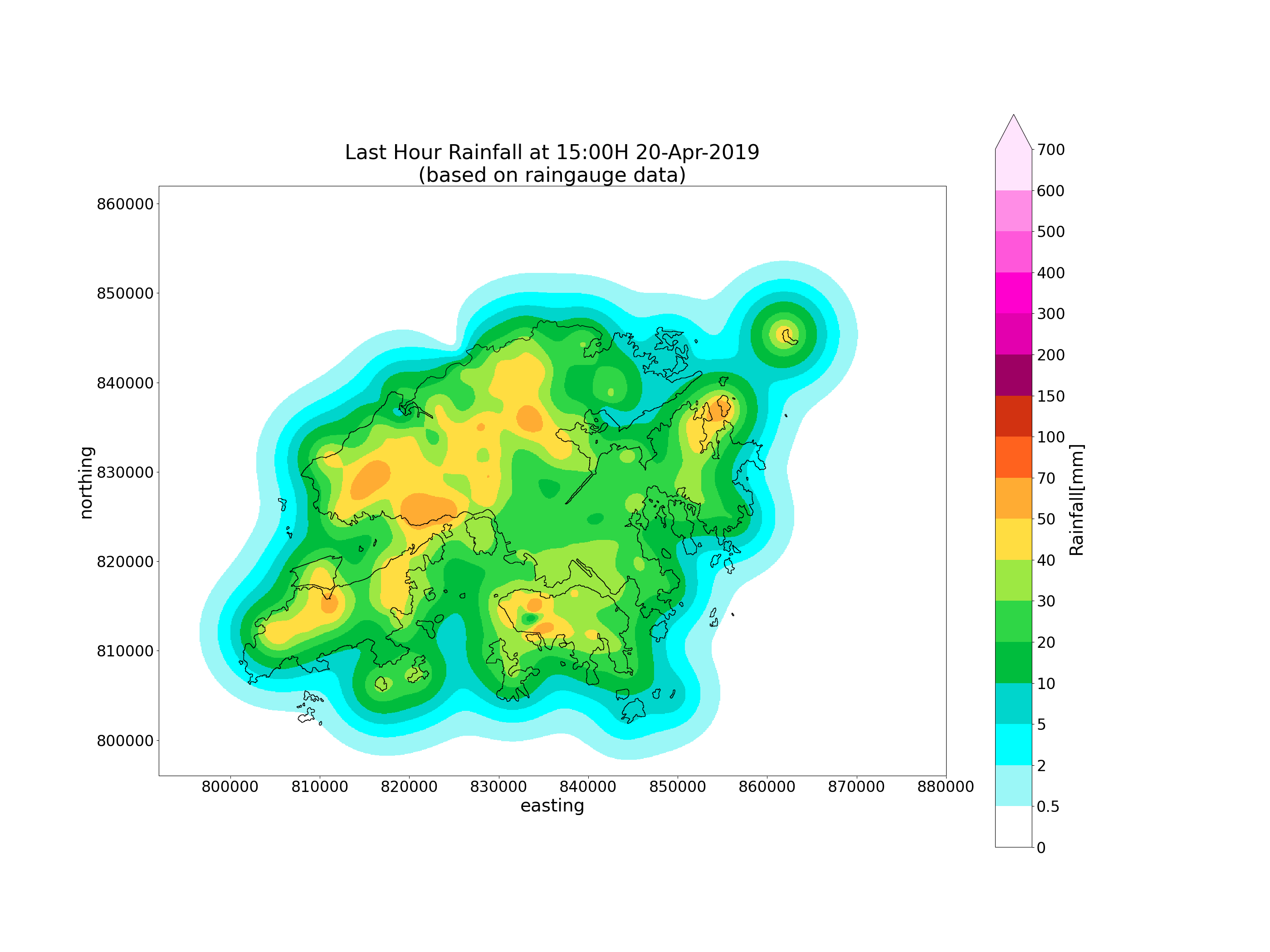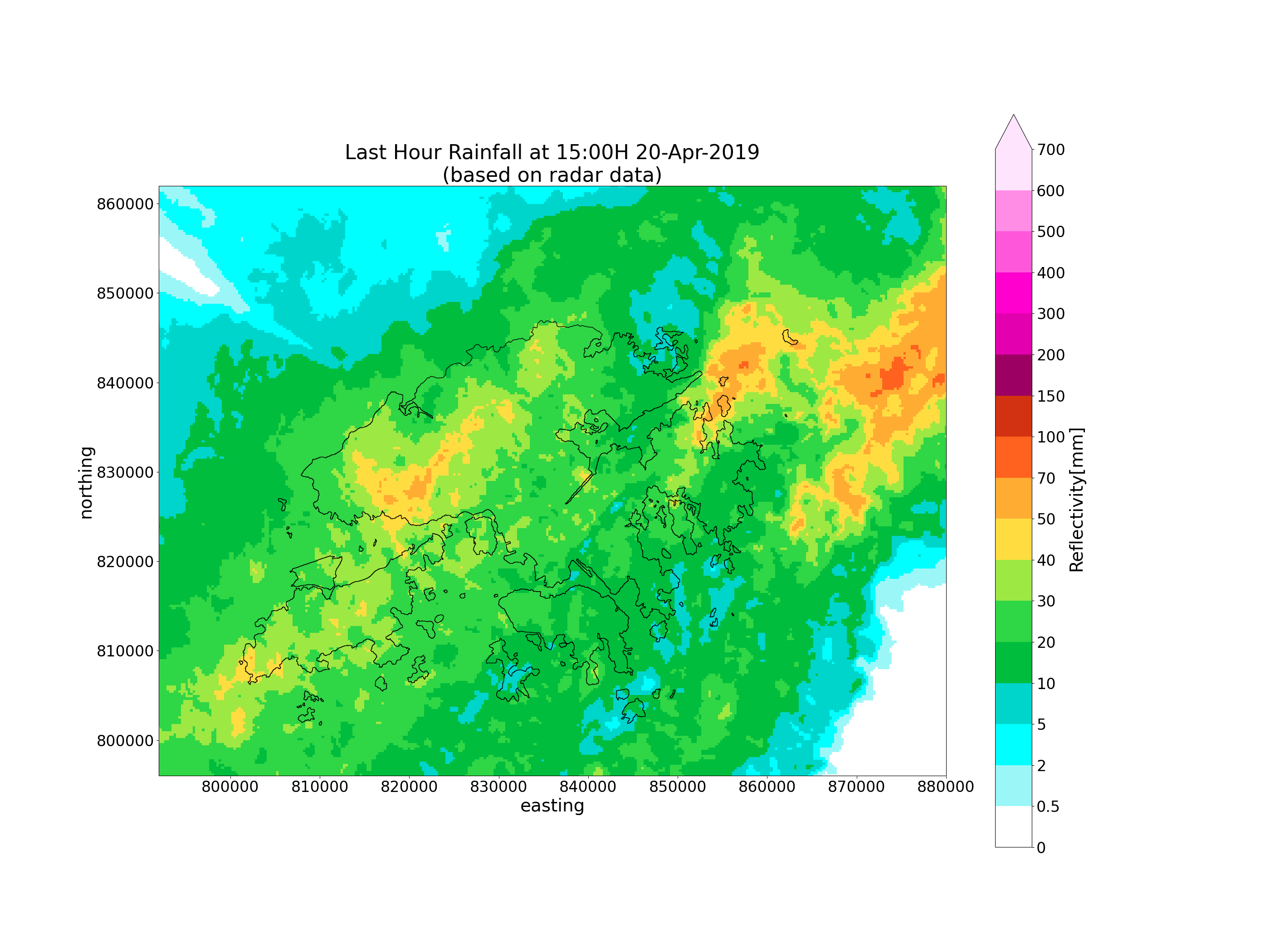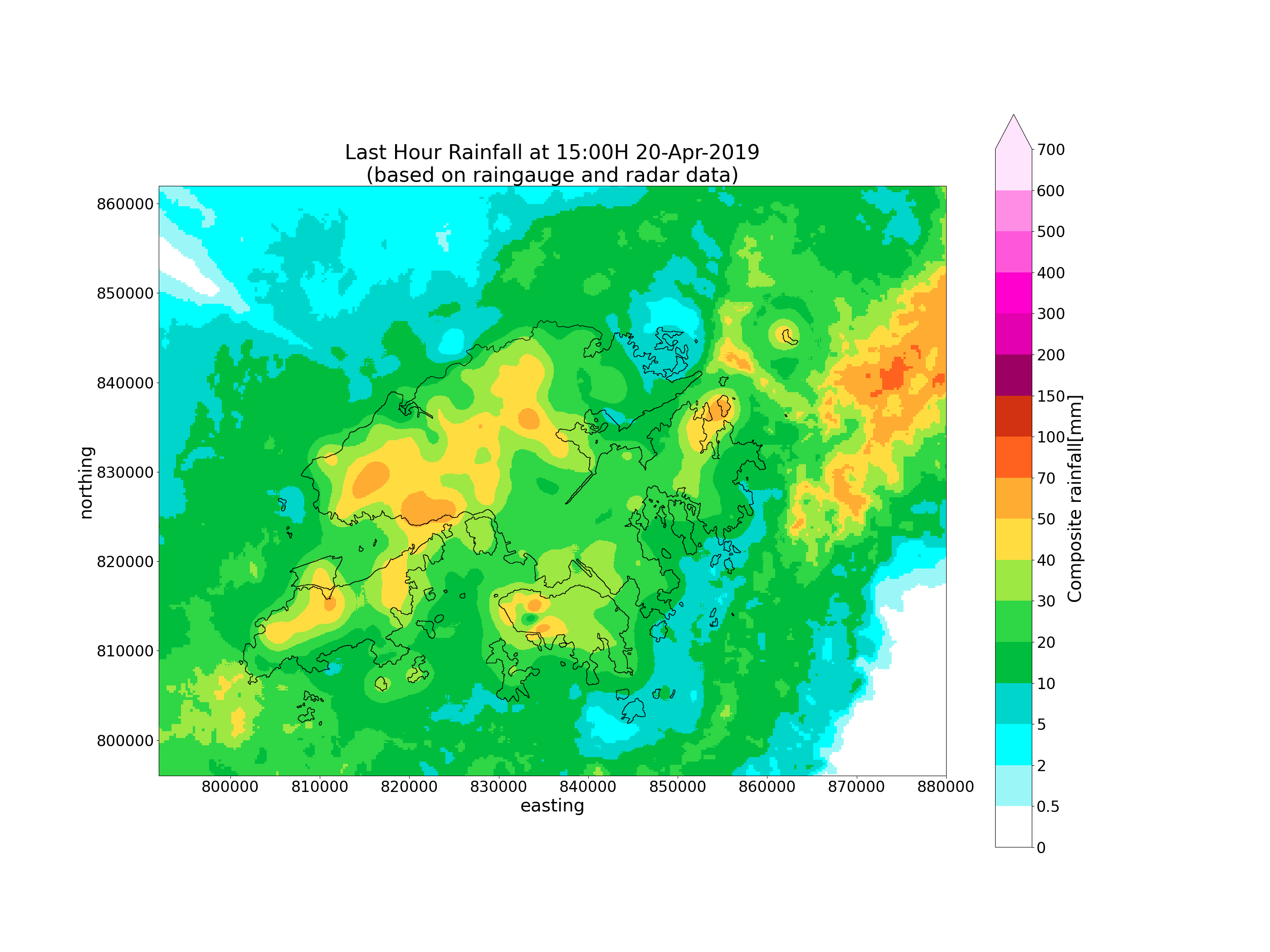# QPE (Hong Kong)¶

This example demonstrates how to perform QPE, using raingauge and radar data from Hong Kong.

## Definitions¶

import os
import time
import tarfile
import numpy as np
import pandas as pd
import copy
import xarray
import scipy
import pyproj
import matplotlib
from matplotlib.colors import BoundaryNorm
import matplotlib.pyplot as plt
from PIL import Image
from pyresample import utils
from sklearn.gaussian_process import kernels

from swirlspy.obs import Rain
from swirlspy.core.resample import grid_resample
from swirlspy.qpe.rfmap import rg_interpolate, comp_qpe, show_raingauge
from swirlspy.qpe.utils import timestamps_ending, locate_file, dbz2rr, rr2rf, \
temporal_interp, acc

plt.switch_backend('agg')
THIS_DIR = os.getcwd()
os.chdir(THIS_DIR)


Out:

## You are using the Python ARM Radar Toolkit (Py-ART), an open source
## library for working with weather radar data. Py-ART is partly
## supported by the U.S. Department of Energy as part of the Atmospheric
## Radiation Measurement (ARM) Climate Research Facility, an Office of
## Science user facility.
##
## If you use this software to prepare a publication, please cite:
##
##     JJ Helmus and SM Collis, JORS 2016, doi: 10.5334/jors.119


## Initialising¶

This section demonstrates extracting raingauge and radar data.

Step 1: Defining an end-time for accumulating rainfall.

acctime = pd.Timestamp('20190420150000').floor('min')
acctime_str = acctime.strftime('%Y%m%d%H%M')


Step 2: Setting up directories for raingauge and radar files.

rad_dir = THIS_DIR + '/../tests/samples/iris/'
rg_dir = THIS_DIR + '/../tests/samples/rfmap/'


Step 3: Generating timestamps and pattern for both radar and raingauge files.

# Timestamps of raingauges
rg_timestrings = timestamps_ending(
acctime + pd.Timedelta(minutes=5),
duration=pd.Timedelta(hours=1),
interval=pd.Timedelta(minutes=5)
)

# Raingauge pattern
rg_pattern = ['rf5m_20'+ts for ts in rg_timestrings]

# Finding time nearest radar file
# to accumulation end time
minute = acctime.minute
nearest_6min = acctime.minute // 6 * 6

acctime_str[:-2]+f'{nearest_6min:02}'
)

duration=pd.Timedelta(hours=1),
interval=pd.Timedelta(minutes=6)
)


Step 4: Extracting raingauge and radar files from their respective directories.

located_rg_files = []
for pat in rg_pattern:
located_rg_files.append(locate_file(rg_dir, pat))



Step 5: Read data from raingauge files into a Rain object. Coordinates are geodetic, following that in the files. There is some noise in the raingauge, so known problematic stations are filtered away.

rg_object_geodetic = Rain(
located_rg_files,
'geodetic',
duration=pd.Timedelta(minutes=5),
NAN=[3276.7, 32767]
)

bad_stations = ['N25', 'SSP', 'D25', 'TWN', 'TMS', 'N14']
)


Step 6: Read radar files into xarray.DataArrays. The data in the files are already in Cartesian Coordinates, in the Centered Azimuthal Projection.

reflec_list = []
file
)

reflec_list.append(reflec)


## Data Reprojection¶

This section demonstrates the reprojection of extracted raingauge and radar data to a user-defined grid.

Step 1: Define the target grid as a pyresample AreaDefinition.

# Defining target grid
area_id = "hk1980_grid"
description = ("A grid centered about Hong Kong with a resolution "
"880m in the x-direction and 660m in the y-direction "
"HK1980 easting/northing coordinates")
proj_id = 'hk1980'
projection = ('+proj=tmerc +lat_0=22.31213333333334 '
'+lon_0=114.1785555555556 +k=1 +x_0=836694.05 '
'+y_0=819069.8 +ellps=intl +towgs84=-162.619,-276.959,'
'-161.764,0.067753,-2.24365,-1.15883,-1.09425 +units=m '
'+no_defs')

x_size = 1000
y_size = 1000

area_extent = (792000, 796000, 880000, 862000)

area_def = utils.get_area_def(
area_id, description, proj_id, projection, x_size, y_size, area_extent
)


Step 2: Convert coordinates of raingauge object to desired projection. In this example, the desired projection is HK1980. This can be achieved by the .reproject() method of the Rain object.

inProj = pyproj.Proj(init="epsg:4326")
outProj = pyproj.Proj(area_def.proj_str)

rg_object = rg_object_geodetic.reproject(inProj, outProj, "HK1980")


Step 3: Regrid radar reflectivity from Centered Azimuthal Projection to HK1980.

reproj_reflec_list = []
for reflec in reflec_list:
reproj_reflec = grid_resample(
reflec,
reflec.attrs['area_def'],
area_def, coord_label=['easting', 'northing']
)
reproj_reflec_list.append(reproj_reflec)

reflectivity = xarray.concat(reproj_reflec_list, dim='time')


## Accumulating and interpolating rainfall¶

Interpolate rainfall recorded by raingauges into the user-defined grid and accumulate radar rainfall over an hour after making the necessary adjustments.

Step 1: Interpolate Rain object to user-defined grid. In this example, ordinary kriging is used.

Using kriging may require further customisation of certain parameters.

# Perform some primitive quality control
upperQ = np.quantile(rg_object.rainfall, .75)
lowerQ = np.quantile(rg_object.rainfall, .25)
iqr = upperQ - lowerQ

noisePos = np.logical_or(rg_object.rainfall > upperQ + 1.5*iqr,
rg_object.rainfall < lowerQ - 1.5*iqr)

alpha = np.where(noisePos, 1e4, 1e-10)

kernel = kernels.Matern()

interpolated_rg = rg_interpolate(
rg_object, area_def, 'ordinary_kriging',
coord_label=['easting', 'northing'],
kernel=kernel,
alpha=alpha,
n_restarts_optimizer=20
)


Step 2: Convert to radar reflectivity to rainrates, interpolate rainrates to times of raingauges, and convert to rainfalls accumulated every 5 minutes.

rainrates = dbz2rr(reflectivity, a=58.53, b=1.56)
# Convert time coordinates of rainrates from start time
# to end time
rainrates_time_endtime = rainrates.copy()
rainrates_time_endtime.coords['time'] = \
[
pd.Timestamp(t) + pd.Timedelta(minutes=6)
for t in rainrates.coords['time'].values
]

rainrates_5min = temporal_interp(
rainrates_time_endtime,
rg_object.start_time + pd.Timedelta(minutes=5),
rg_object.end_time,
intvl=pd.Timedelta(minutes=5),
)
rainfalls = rr2rf(rainrates_5min, scan_duration=5)


Step 3: Accumulate rainfall over an hour.

acc_rf = acc(
rainfalls,
rg_object.end_time,
acc_period=pd.Timedelta(minutes=60)
)


## Compositing rainfall¶

Perform compositing on radar and raingauge derived rainfall to obtain a composite QPE.

Some parameter tuning may be required to make the observations fit better with each other.

comprf = comp_qpe(
area_def,
rg_object=rg_object,
rg_interp=interpolated_rg,
)


## Plotting¶

Plot composited radar and raingauge rainfall.

# Plotting function for neatness.

def plot_map(
da, rg_object, acctime, area_def,
savepath='',
):

"""
A custom function for plotting a map.

Parameters
--------------
da: xarray.DataArray
Contains data to be plotted.
rg_object: Rain
Contains raingauge data.
acctime: pd.Timestamp
Contains the endtime of the accumulation
period.
based: str
Type of data plotted in the map.
savepath: str
Path to save the image to.

"""
# Defining the colour scheme
levels = [
0, 0.5, 2, 5, 10, 20,
30, 40, 50, 70, 100, 150,
200, 300, 400, 500, 600, 700
]

cmap = matplotlib.colors.ListedColormap([
'#ffffff', '#9bf7f7', '#00ffff', '#00d5cc', '#00bd3d', '#2fd646',
'#9de843', '#ffdd41', '#ffac33', '#ff621e', '#d23211', '#9d0063',
'#e300ae', '#ff00ce', '#ff57da', '#ff8de6', '#ffe4fd'
])

norm = BoundaryNorm(levels, ncolors=cmap.N, clip=True)

# Plotting axes
plt.figure(figsize=(28, 21))
crs = area_def.to_cartopy_crs()
ax = plt.axes(projection=crs)

# Plot data
cmap=cmap,
norm=norm,
extend='max',
cbar_kwargs={'ticks': levels, 'format': '%.3g'}
)

cb.ax.set_ylabel(
da.attrs['long_name']+'['+da.attrs['units']+']',
fontsize=28
)

cb.ax.tick_params(labelsize=24)

# Setting labels
ax.xaxis.set_visible(True)
ax.yaxis.set_visible(True)

for tick in ax.xaxis.get_major_ticks():
tick.label.set_fontsize(24)
for tick in ax.yaxis.get_major_ticks():
tick.label.set_fontsize(24)

ax.xaxis.label.set_size(28)
ax.yaxis.label.set_size(28)

# Coastlines
shpfile = THIS_DIR + \
for record, geometry in zip(shp.records(), shp.geometries()):

# Show title
plt.title(
(f"Last Hour Rainfall at {acctime.strftime('%H:%MH %d-%b-%Y')}\n"
f"(based on {based} data)"),
fontsize=32
)

plt.savefig(savepath, dpi=300)

# Plotting maps

# Raingauge only
plot_map(
interpolated_rg, rg_object,
acctime, area_def,
based='raingauge',
savepath=THIS_DIR+f'/../tests/outputs/raingauge_{acctime_str}.png'
)

plot_map(
acc_rf, rg_object,
acctime, area_def,
)


•••